# Multiplexer and De-Multiplexer

## Multiplexer and De-Multiplexer

In the following article we will discuss the construction and operataion of multiplexer and de-multiplexer in detail.

### The Multiplexer (MUX)

The operation of sending one or more analog or digital signals over a common transmission line at different times or speeds is known as multiplexing,  and the device used to do this is known as Multiplexer.

The multiplexer, shortened to “MUX”  is a combinational logic circuit designed to switch one of several input lines through to a single common output line by the application of a control signal.

Multiplexers or MUX’s, can be either digital circuits made from high speed logic gates used to switch digital or binary data or they can be analog types using transistors, MOSFET’s or relays to switch one of the voltage or current inputs through to a single output.

The most basic type of multiplexer device is that of a one-way rotary switch as shown below:

### Basic Multiplexing Switch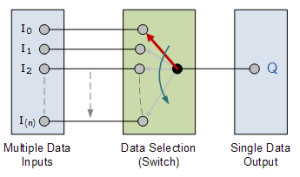Fig.1

The rotary switch is a manual switch that can be used to select individual data or signal lines simply by turning its inputs “ON” or “OFF”.

In digital electronics, multiplexers are constructed from individual analog switches encased in a single IC package as compared to the “mechanical” type selectors such as normal conventional switches and relays.

Generally, the selection of each input line in a multiplexer is controlled by an additional set of inputs called control lines and according to the binary condition of these control inputs, either “HIGH” or “LOW” the appropriate data input is connected directly to the output.

Normally, a multiplexer has an even number of 2N data input lines and a number of “control” inputs that correspond with the number of data inputs.

We can build a simple 2-line to 1-line (2-to-1) multiplexer from basic logic NAND gates as shown.

### 2-input Multiplexer Design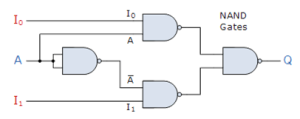Fig.2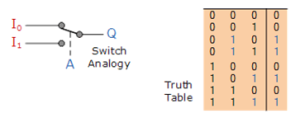Fig.3

The input A of this simple 2-1 line multiplexer circuit acts to control which input ( I0 or I1 ) gets passed to the output at Q.

From the truth table we can see that when data select input, A is LOW (logic 0), input I1 passes its data to the output while input I0 is blocked.

When data select A is HIGH (logic 1), input I0 is passed to Q while input I0 is blocked.

In this above example, the 2-input multiplexer connects one of two 1-bit sources to a common output, producing a 2-to-1-line multiplexer and we can confirm this in the following Boolean expression.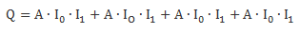and for  2-input multiplexer circuit above, this can be simplified to:Larger multiplexer circuits can be implemented using smaller 2-to-1 multiplexers as their basic building blocks.

So for a 4-input multiplexer we would require two data select lines as 22 =4, hence two data control lines will give a circuit with four inputs.

### 4-to-1 Channel Multiplexer

A 4-1 MUX with four inputs: A, B, C, D and two data select lines  a and b is  shown below: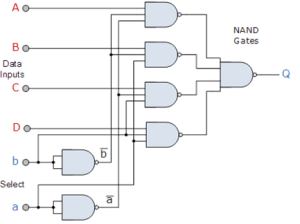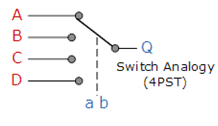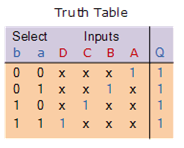Fig.4

The Boolean expression for this 4-to-1 Multiplexer above with inputs A to D and data select lines a, b is given as:

Q = abA + abB + abC + abD

In this example at any instant of time only ONE of the four analogue switches is closed, connecting only one of the input lines A to D to the single output at Q.

As to which switch is closed depends upon the addressing input code on lines “a” and “b“, so for this example to select input B to the output at Q, the binary input address would need to be “a” = logic “1” and “b” = logic “0”.

### Multiplexer Input Line Selection

The selection of the data through the multiplexer can be shown as a function of the data select bits as below: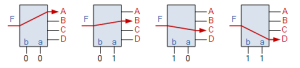Fig.5

Adding more control lines will allow the multiplexer to control more inputs but each control line configuration will connect only ONE input to the output.

### 4-1 MUX using Logic Gates

The implementation of the Boolean expression of the 4-1 MUX,  using seven individual gates consisting of AND, OR and NOT gates is shown below:

Fig.6

### Multiplexer Symbol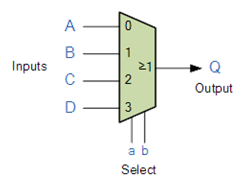Fig.7

### The Demultiplexer

The Demultiplexer or “Demux” for short, is the exact opposite of the Multiplexer.

The demultiplexer takes one single input data line and then switches it to any one of a number of individual output lines one at a time.

The demultiplexer converts a serial data signal at the input to a parallel data at its output lines as shown below.

### 1-to-4 Channel De-multiplexer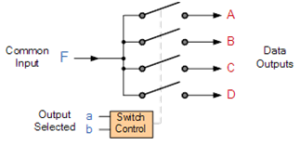Fig.8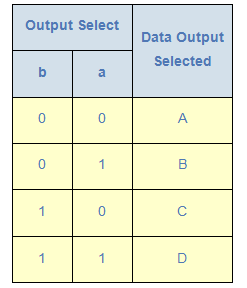Fig.9

The Boolean expression for this 1-to-4 Demultiplexer above with outputs A to D and data select lines a, b is given as:

F = abA+ abB + abC+ abD

### Demultiplexer Output Line Selection

The function of the Demultiplexer is to switch one common data input line to any one of the 4 output data lines A to D in our example above.

As with the multiplexer the individual solid state switches are selected by the binary input address code on the output select pins “a” and “b” as shown below: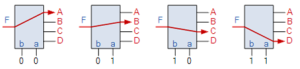Fig.10

### 1-to-4 Demultiplexer using Logic Gates

The implementation of the Boolean expression above using individual logic gates would require the use of six individual gates consisting of AND and NOT gates as shown.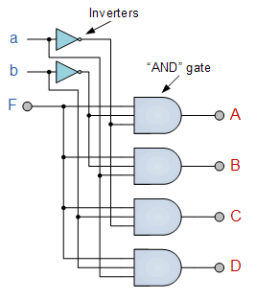Fig.11

### The Demultiplexer Symbol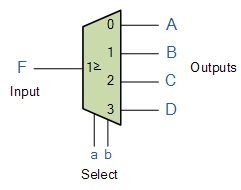Fig.12